## LaTeX offers features to automatically generate a table of contents, a list of figures and a list of tables. Learn here how to use them.

Generating a table of contents can be done with a few simple commands. LaTeX will use the section headings to create the table of contents and there are commands to create a list of figures and a list of tables as well. I will give a small example code to create a table of contents first:

\documentclass{article}

\begin{document}

\tableofcontents
\newpage

\section{Section}

Dummy text

\subsection{Subsection}

Dummy text

\end{document}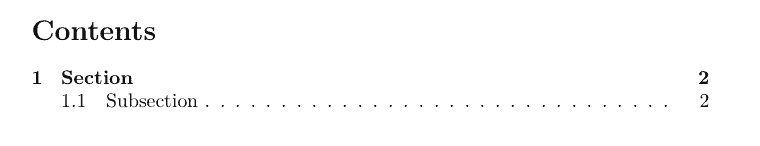## List of figures / tables

The generation of a list of figures and tables works the same way. I added a dummy figure and table and put the lists in the appendix of my document:

\begin{document}
...
\begin{figure}
\caption{Dummy figure}
\end{figure}

\begin{table}
\caption{Dummy table}
\end{table}
...
\begin{appendix}
\listoffigures
\listoftables
\end{appendix}

\end{document}

After compiling two times again, the lists will be generated like this: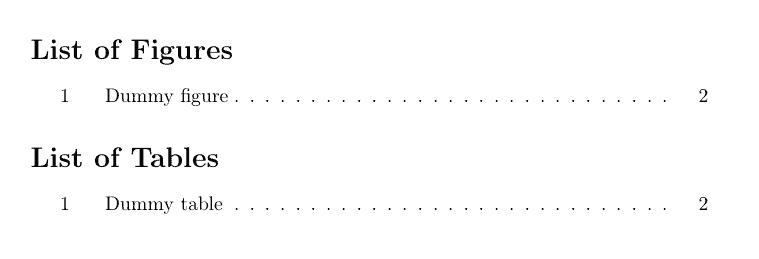## Depth

Sometimes it makes sense to only show a subset of the headings for all sections or for a particular section. For this reason you can set a the tocdepth by using the command \setcounter{tocdepth}{X}, where X is the desired depth. A value of 0 means that your table of contents will show nothing at all and 5 means, that even subparagraphs will be shown. The value has to be set in the preamble of your document and automatically applies to the whole document:

% ...

\setcounter{tocdepth}{1} % Show sections
%\setcounter{tocdepth}{2} % + subsections
%\setcounter{tocdepth}{3} % + subsubsections
%\setcounter{tocdepth}{4} % + paragraphs
%\setcounter{tocdepth}{5} % + subparagraphs

\begin{document}
%...
\tableofcontents
%...
\end{document}

Using the example from above, the setting tocdepth = 1 will lead to the following output: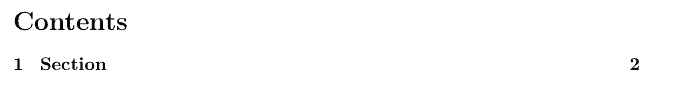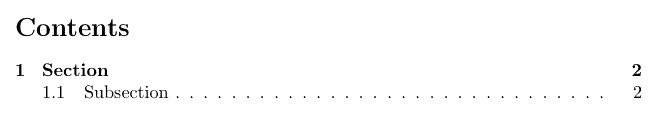If you don’t want to change the depth for all sections, you can also adjust the tocdepth for each section individually. In this case you don’t have to set the tocdepth before the section which should have more or less depth.

%...
\begin{document}
%...
\addtocontents{toc}{\setcounter{tocdepth}{1}} % Set depth to 1
\section{Another section}
\subsection{Subsection}
\subsubsection{Subsubsection}
%...
\addtocontents{toc}{\setcounter{tocdepth}{3}} % Reset to default (3)
\end{document}

This will generate the following table of contents, using the default tocdepth for the first section, but tocdepth = 1 for this section: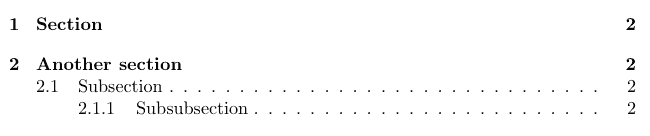## Spacing

%...
\usepackage{setspace}
%...
\begin{document}
%...

%...
\begin{document}
%...
\doublespacing
\tableofcontents
\singlespacing
%...

Which will lead to the following output: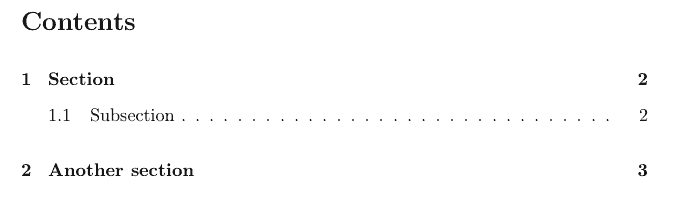## Summary

• Autogenerate a table of content using \tableofcontents
• Create lists of your figures and tables with \listoffigures and \listoftables
• Always compile twice to see the changes
• Globally change the depth with \setcounter{tocdepth}{X}; X = {1,2,3,4,5}